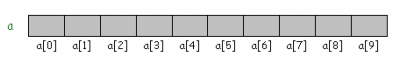# Using Single Dimension Arrays in C++

An array in general is a list or a series of objects or elements of same data type and all of the elements are attached to each other in memory locations. You only need to use indexes or references to all of the fields. Arrays are very useful in any programming language as they can be used to create linked lists, stacks and many other types of data structures. To master any programming language it is necessary to have command on its important elements. This is why I am going to write this step by step guide for beginners and I will show you how you can initialize a single dimensional array and how you can access the values of it.

### Instructions

• 1

Declaring an array: To declare an array which is shown in figure the simple line of code is:

int a;

Above line will initialize a single dimensional array which has 10 integer type elements and all of them have different index e.g. the index number of 6th element is 5.• 2

Initializing an Array:

If you want to initialize the elements of an array then it is very simple, just use the following line of code to initialize the values.

int a   = {1,4,5,1,5,8,3,7,1,5};

Now starting from left to right in braces first value will be stored to a, second to a and so on.

This ways you can initialize a single dimensional array.

• 3

Accessing the values:

To access the value of a specific element in an array we use the index of that element and name of the array for example if you want to pass value present at index 2 to some integer variable then you can do it like this:

int temp  =  a;

Above statement will access the value in array a present at index 2 and will copy that to temp variable.

You can also set the value of an element by using following line:

a = 19;

Here integer value 19 will be copied in array at index 5, previous value will be modified.

• 4

Example code:

#include

using namespace std;

int arr = {1,2,3,4,5,6};

int main ( ) {

for (int i = 0 ; i<=4; i++ )

{

cout<<”Array vaue at index ”<< i << “is  =  ”<< arr[i] <

}

Return 0;

}

•